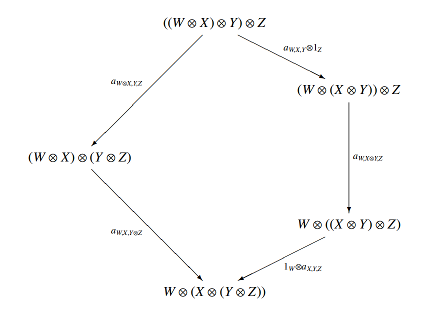# How many ways can you parenthesize an expression?

Mathematical diagrams are usually rectangles, sometimes triangles. So pentagonal diagrams are odd.

Here’s a pentagonal diagram from a paper by John Baez and Mike Stay. Why is it a pentagon?There are five ways to parenthesize an expression with four terms, and the diagram is showing how all ways of parenthesizing four terms are related.

How do we know that there are five ways to parenthesize four terms? We could list them, but then how do we know we didn’t leave out a possibility?

It turns out that the number of ways to parenthesize an expression with n+1 terms is Cn, the nth Catalan number. An expression for the Catalan numbers can be derived by starting with a recurrence relation that the numbers must satisfy and then producing their generating function. The end result is the following identity:If we set n = 3, we get C3 = 5, confirming that there are five ways to parenthesize four terms.

The Catalan number pop up in many applications. For example, Richard Stanley has posted a 96-page PDF file of combinatorial problems whose solution involves Catalan numbers.

Here’s an odd observation regarding Catalan numbers. If Cn is an odd Catalan number, then n = 2k – 1. And it appears that if k ≥ 9, the last digit of Cn is always 5, though this has not been proven.

## One thought on “How many ways can you parenthesize an expression?”

1. All such parenthesizations are even parametrized by a famous polytope, the associahedron (or the Stasheff polytope), which has a rich combinatorial structure.